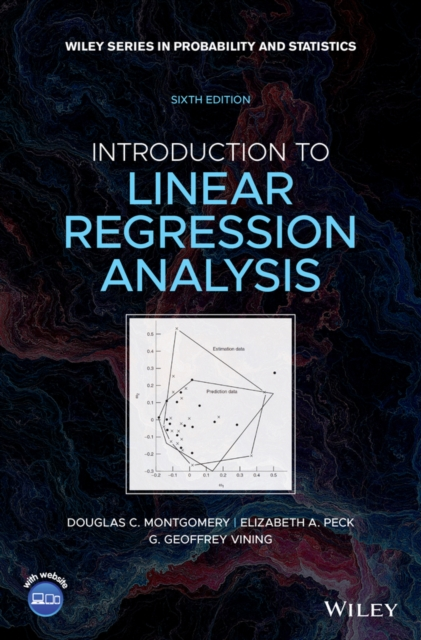# Introduction to Linear Regression Analysis, 6th Edition Hardback

Hardback

• Information

#### Description

INTRODUCTION TO LINEAR REGRESSION ANALYSIS A comprehensive and current introduction to the fundamentals of regression analysis Introduction to Linear Regression Analysis, 6th Edition is the most comprehensive, fulsome, and current examination of the foundations of linear regression analysis.

Fully updated in this new sixth edition, the distinguished authors have included new material on generalized regression techniques and new examples to help the reader understand retain the concepts taught in the book.

The new edition focuses on four key areas of improvement over the fifth edition: New exercises and data sets New material on generalized regression techniques The inclusion of JMP software in key areas Carefully condensing the text where possible Introduction to Linear Regression Analysis skillfully blends theory and application in both the conventional and less common uses of regression analysis in today's cutting-edge scientific research.

The text equips readers to understand the basic principles needed to apply regression model-building techniques in various fields of study, including engineering, management, and the health sciences.

£133.00

£102.18